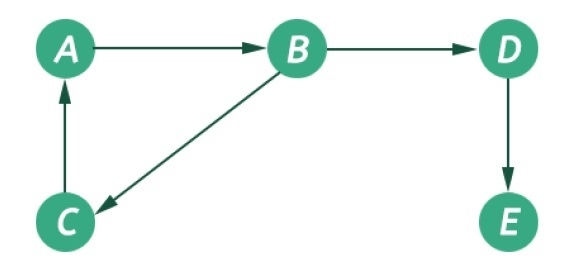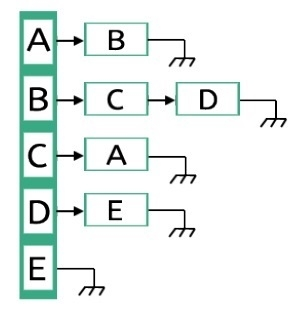# Adjacency lists in Data Structures

The graph is a non-linear data structures. This represents data using nodes, and their relations using edges. A graph G has two sections. The vertices, and edges. Vertices are represented using set V, and Edges are represented as set E. So the graph notation is G(V,E). Let us see one example to get the idea.In this graph, there are five vertices and five edges. The edges are directed. As an example, if we choose the edge connecting vertices B and D, the source vertex is B and destination is D. So we can move B to D but not move from D to B.

The graphs are non-linear, and it has no regular structure. To represent a graph in memory, there are few different styles. These styles are −